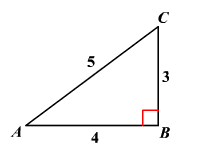# Cosine

The cosine of an angle is the trigonometric ratio of the adjacent side to the hypotenuse of a right triangle containing that angle.

Example:

In the triangle shown, $\mathrm{cos}\left(A\right)=\frac{4}{5}$ and $\mathrm{cos}\left(C\right)=\frac{3}{5}$The cosine ratio is the same regardless of the size of the right triangle. So, it is often easiest to consider a right triangle with a hypotenuse of length $1$ .

The cosine ratio can also be thought of as a function, which takes different values depending on the measure of the angle. You can measure an angle in degrees or radians .

The reciprocal of the cosine ratio is known as secant ratio of an angle, abbreviated as "sec".

That is,

.

In the above example, $\mathrm{sec}\left(A\right)=\frac{5}{4}$ and $\mathrm{sec}\left(C\right)=\frac{5}{3}$ .